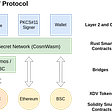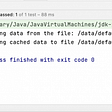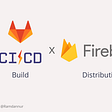# Working with Rational numbers

Or how to write something when you just don’t know what to do :)

There are times. There are times in our lives when we don’t know what to say. When we think that we stopped in one place. Hell, like we not solving anything new. Actually nothing wrong with that. It is hard to invent something new and clever these days.

# The prologue

The year 2021, I was just an engineer in some company. And I received an offer from the other big company. I was really happy and accepted it. But due the covid the document processing took a while, so it was unknown, when I will start. Yet people said that July should be fine, we have some time.

Since I lived in Dubai and my contract came to the end, I decided to terminate everything, come back to my country and wait there. Without job. Only with earned money, that I didn’t want to spend, since I would need them later. Without knowing, when I will move in. With stuff left in Dubai in paid storage…

Strange decision right? Yet I made it. Similarly in java we have a float and double types. Those types aren’t new, and were created many many years ago. According to wiki, it was there since Fortran language.

We know those famous Java Puzzlers, when `2.00–1.10` gives you not `0.9` but `0.89999999` number. You can read more about representation convention and how discrete float numbers works here.

The main point, is that Float/Doubles are clever rearrangement of bits, that were previously used for Int/Long. And this rearrangement gave an ability to work with divisions, square roots and other calculations, that involve not integer numbers. Yet it had its limits in precision. You can read more about how floating point numbers were borned in the book Code: The Hidden Language of Computer Hardware and Software.

When I read about all of that, I thought “why not to create” really Rational type, so we will be able to have numbers, like `18/396` which is `0.0(45)` in decimal representation. Compare to it, float number will look like `0.045454547`.

Same as my decision. Wierd and strange. Same as this prologue. Useless. But interesting, how it will go :)

# Basic ops

Simple an basic operations were easy to add:

As my decision this part was really simple. Simple and premature, as I faced eventually with understanding of few things. Things that I don’t know how to solve (mathematically and with java/kotlin):

1. There will be a lot of repeating numbers. `1/2`, `2/4`, `100/200 `— all of them are in fact the same number `0.5`.
2. Both denominator and numerator can be negative, which makes `(-1)/2` and `1/(-2)` the same number.
3. For instance min float number is `1.4E-45`, and my min rational number will be `4.7E-10`. Max float number is `3.4E38` whic my max rational number is just `2.1E9`. So float has much bigger spread, than presented rational number
4. In the same time my rational number is twice bigger than float. 8 bytes versus just 4 bytes

Now you understand, how useless this class is. Yet, it was still interesting to create it. So let’s continue.

# Canonical method

## Aim

As I said before there were different repeating numbers. For instance, `1/2`, `10/20`, `10000/20000` were the same number `0.5`. So I wanted to have method, that would convert any rational number to its canonical form (minimal numerator and denominator with preserving the value).

To receive it, I needed to know the greatest common divisor of both numbers, and then divide them both by it.And here I discovered binary GCD.

## Binary GCD

If you will try to solve the GCD problem, the classical solution will be this one (it can be recursive or iterative, I presented recursive, since it’s more concise).

But I was interested to find more fast approach. And I found binary GCD algorithm. I will not dive into details of explanation and will just present the code, since I used it inside `Rational`.

## Comparison with other GCD by benchmarks

Well, let’s check: is it really faster than recursive approach. I will also include iterative approach (let’s call it `loopGcd`) to be more fair. You may ask, why you do this for kotlin or java, maybe it is better to check in more low level languages? Probably you right, but I was just curious :)

Let’s take a simple benchmark for different amount of pairs (numbers of which we will take a GCD). Also let’s test it for repeating the same calculation and using different numbers every time.

Here is a snippet of benchmark:

Now let’s see the result:

As you can see for small amount of numbers there is no real difference between recursive, loop and binary gcd. However on a big amount of numbers binary GCD is winning.

I would say that if you not using native code for building gcd algorithm, you can use any of them.

# Formatting

Now the time is to print our rational number. For instance for number `18/99` we want to print `0.(18)`, which means `0.1818181818...`.

To do this, we need to do the two main steps.

## Split on an array of digits

First one, we need to build a decimal representation of our rational number. Let’s define `Decimal` nested class:

It contains of:

• sign (`signum`property, `1` or `-1` respectively)
• array of digits (`digits` property)
• amount of digits after the dot, as decimal part (`scale` property)
• amount of digits inside bracets, repeating part (`repeat` property)

To formulate the instance of such class, we need to do a good old school division:

This method can be basically several parts:

1. In the first part we try to find, do we have the whole part of the number or not. If yes, then we split that whole part on array of digits. It not, then we add just 0
2. In the second part we divide as in school. We take a dividend. If it less than divisor, we multiply it by ten until, it will be greater (in the same time we add zeroes to our digits array). After that we compute the whole part of division (it will be always a digit between 1 and 9) and check the remain. If remain is zero, we completed the division. If not, then repeat.
3. The second step can be infinite in time in case of rational numbers like `1/3` with infinite count of numbers in decimal part. So we keep all remaining in the hash table and check it on each step. If on some step we understand, that our remain already contains in the table, then we found repeating part of our decimal representation, and we completed the division.

## Format

Now the time to format our number, giving the information, provided by `Decimal`.

If we want to have a deal with really big numbers, using `Int` for `numerator` and `denominator` might be not enough. In that case would be nice to use `BigInteger`.

Well, we could just change everything to use such type, but why not to have a two types, one for small numbers and one for big (like `Int` & `BigInt`).

## Basic: The Interface

Let’s make an interface first, that will generalize work with numbers. Later you will see, why such interface will be useful:

We have pretty much the same methods, as for our initial class (now it will be called `OldRational`)

Now we have a decision:

• Either we want to keep two separate implementation repeating the all calculations but for different types (because there is no common interface for `Int` & `BigInteger` in kotlin that unite them by math operations).
• Or we want to try to avoid this duplication

As I said, since the whole this solution is meaningless and just a mental experiments, let’s follow the second approach.

## Number wrapper

Actually all the operations are in idea the same. If you want to multiply two rational numbers, you multiply the numerators and denominators, regardlessly of their concrete type. If you want to convert rational to decimal representation, you just need to know how to divide numerator and denominator and how to convert them to array of digits. You don’t care if it `Int` or `BigInteger` type.

So we just need to abstract away from concrete types and think about numbers as some general type. To do that, let’s introduce the next interface:

So we have our custom number interface. Having it, we establish a typed protocol “we know and sure that all numbers can be added/subtracted and etc by their type”. For instance, all you can add integer to interger, or long to long, but not long to int. Pretty much similar to kotlin type system, hah?

Now we can have two implementation of numbers:

Now it is type to basically generalize everything (almost):

## Decimal Computer

I said almost, because I didn’t include formulating of `Decimal` number here, since it deserve the separate section. Instead we will build a computer, that can calculate decimal for some `Number<N>` and will provide a different implementations for `BigInteger` and for `Int`.

Since the `Number` actually can be anything, we don’t know in general what `zero` and `ten` means, that why they will be specified by children.

The implementation for `BigInteger` looks pretty straightforward:

With `Int` it is a bit more complicated. You see, then int can be overflowed, so this line:

`var remain: N = numerator % denominator * ten`

For instance if `numerator = 1000000000` and `denominator = Int.MAX_VALUE` , then `numerator` will be less than `denominator` and the remain of division will be equal to `numerator`. However multiplication on ten will give the value which is more than `Int.MAX_VALUE`. To fix that, `remain` should be the `LongNumber` type not `IntNumber`.

To achieve that we will use Proxy pattern and a bit of hack.

So every time we want to `compute` two int numbers, we convert them first to long, and then do calculations for long values.

# Canonized Rational

We have two types of `Rational` interface: for handling small numbers and big numbers.

Suppose now that we want to convert our rational number to canonic form each time, we finished our calculation. It will help us to keep numerator and denominator small enough if that’s possible.

But with what we have now, we need to remember to call `canonical` method after each operation. Would be nice to automate it. And in the same time we don’t want to have two more types: `SmallCanonizedRational` & `BigCanonizedRational`. Suppose in the future we will have one more implementation of `Rational` interface. We will have to remember to provide another canonized type for it, too. And here when Decorator Pattern shines.

We just wrap any `Rational` number with decorator that invoke `canonical` after each operation. We also use a trick with companion object to imitate `CanonizedRational` constructor calling, but instead we call factory method `invoke` that converts wrapped rational number to its canonical form.

# Afterwords

As I said multiple times through the article, I’m not sure there is a real usage of what we have done. This post was more mind game “Let’s try to do this”.

Moreover it also showed how far we can go with generalization and overengineering in attempt to achieve DRY principle. And even if Rational numbers would be needed somewhere in prod. I’m not sure that you need to build whole this hierarchy for that. Maybe sometimes you can sacrifice a duplications a bit, in order to keep your structure clean and simple (according to KISS principle).

The balance matters in the end!

If you liked that article, don’t forget to support me by clapping. If you want to support me more, just subscribe to my blog and keep an eye on new articles. Also, if you have any questions, comment me, and let’s have a discussion. Happy coding!

Also, there are other articles, that can be interesting:

--

--

--

## More from Michael Spitsin

Love being creative to solve some problems with an simple and elegant ways

Love podcasts or audiobooks? Learn on the go with our new app.

## Explaining XDV Protocol## Sensitive information can be leaked from Trello dashboards## const yearInReview = new Date(2020);## Hackerrank jumping on the clouds solution## Dealing with Java Exceptions in a functional way## When is writing code documentation worthwhile## Michael Spitsin

Love being creative to solve some problems with an simple and elegant ways

## Facade Design Pattern in Kotlin## Android CI/CD Implementation with Gitlab and Firebase## Observer pattern for loose coupling